# Electronics and Communication Engineering - Digital Electronics

11.

If an analog voltage is expressed in binary using 4 bits, each successive binary count would represent

 A.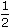of total voltage B.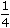of total voltage C.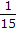of total voltage D.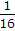of total voltage

Answer: Option C

Explanation:

Since 1111= decimal 15, each successive binary count represent 1/15 of total voltage.

12.

In a 4 input AND gate, the total number of High outputs for 16 input states are

 A. 16 B. 8 C. 4 D. 1

Answer: Option D

Explanation:

In only one combination are all inputs high.

13.

Symmetrical square wave of time period 100 μs can be obtained from square wave of time period 10 μs by using

 A. divide by 5 circuit B. divide by 2 circuit C. divide by 5 circuit followed by divide by 2 circuit D. BCD counter

Answer: Option C

Explanation:

Frequency has to be divided by 10.

14.

The Boolean expression for the shaded area in the Venn diagram is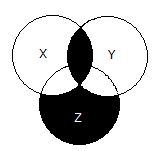A. X + Y + Z B. XY + Z + X YZ C. X Y Z + XY D. X + Y + Z

Answer: Option C

Explanation:

No answer description available for this question. Let us discuss.

15.

The initial state of a Mod-16 counter is 0110. After 37 clock pulses the state of counter will be

 A. 1011 B. 0110 C. 010 D. 0001

Answer: Option A

Explanation:

After 37 clock pulses the state will be the same as after (37 - 32), i.e., 5 clock pulses. 6 + 5 = 11. Hence 1011.

#### Current Affairs 2021

Interview Questions and Answers